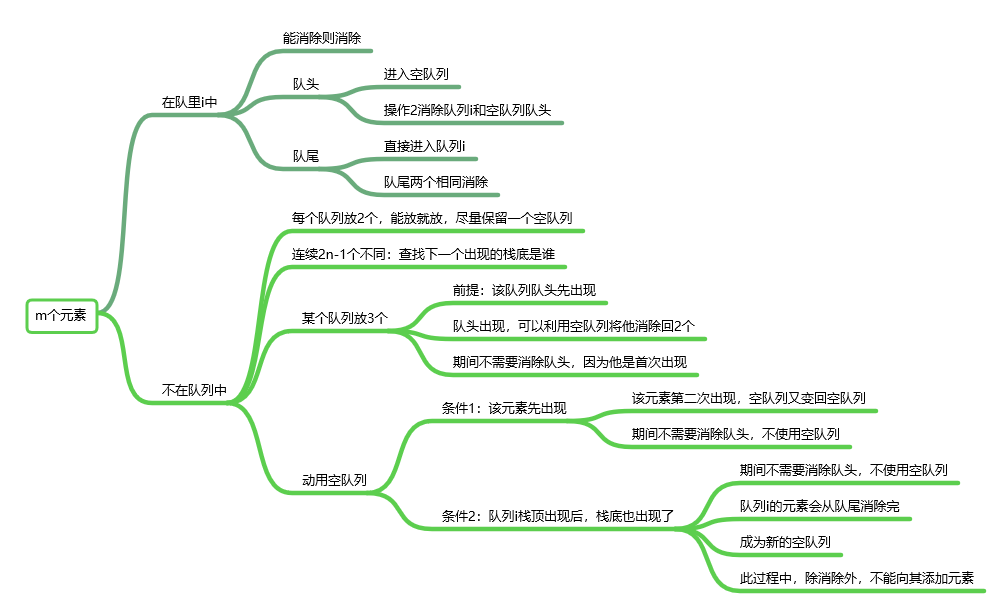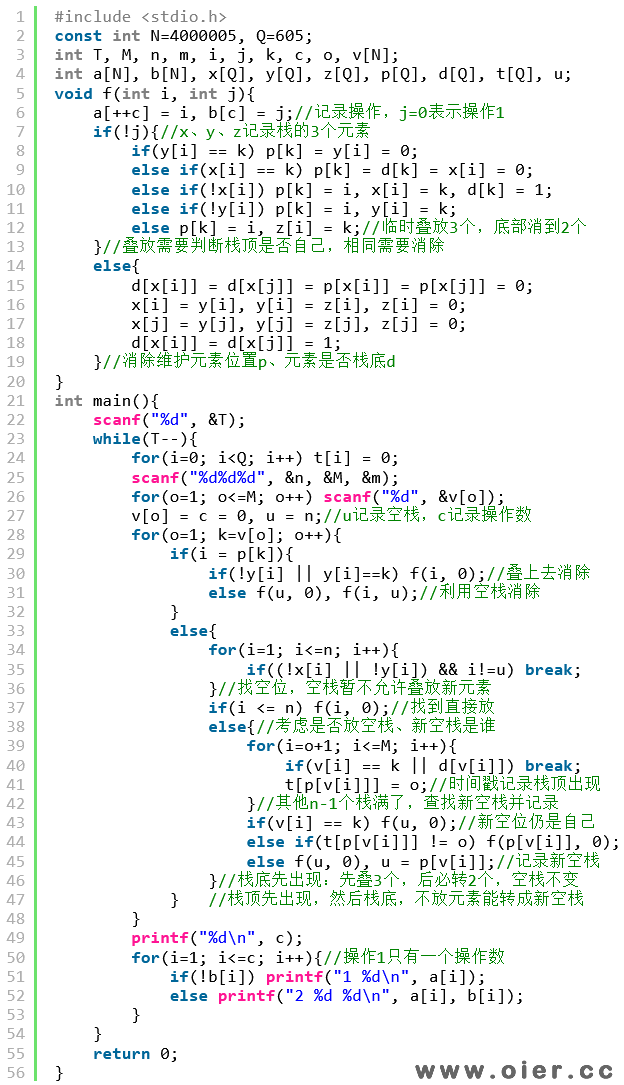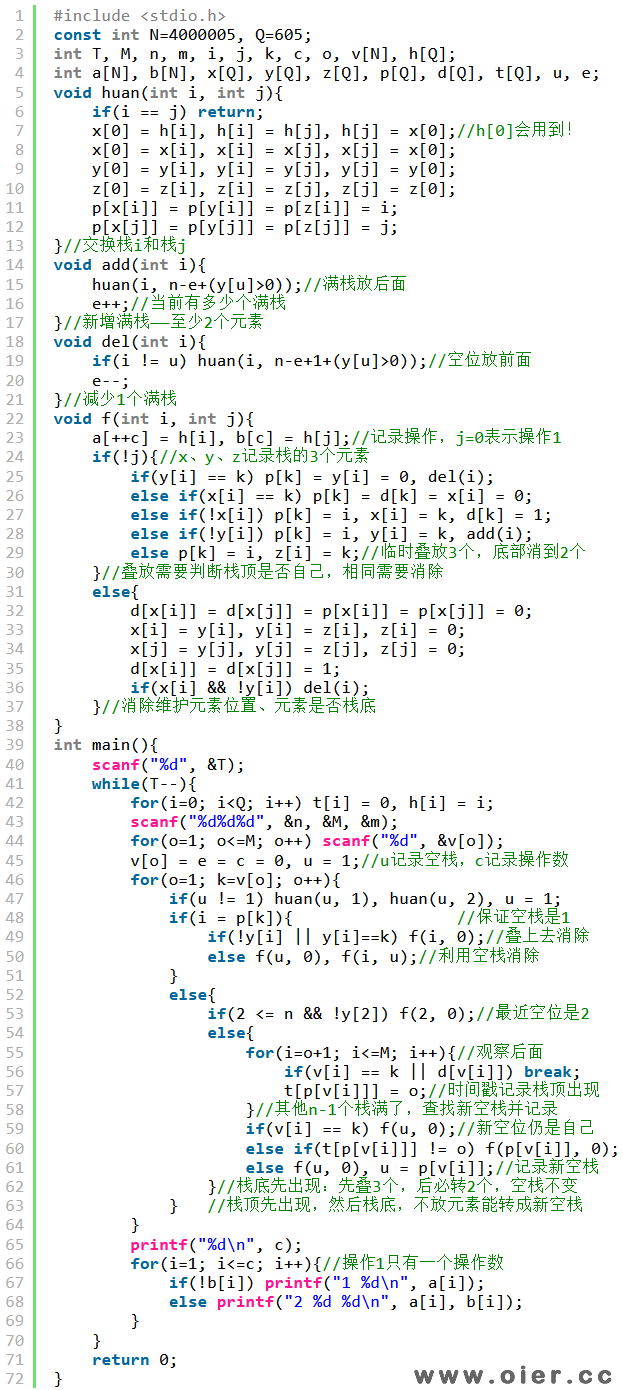438+

## 题目描述

– 选择一个栈，将牌堆顶上的卡牌放入栈的顶部。如果这么操作后，这个栈最上方的两张牌有相同的图案，则会自动将这两张牌消去。
– 选择两个不同的栈，如果这两个栈栈**底**的卡牌有相同的图案，则可以将这两张牌消去，原来在栈底上方的卡牌会成为新的栈底。如果不同，则什么也不会做。

## 输入输出样例

### 输入样例 #1

1
2 4 2
1 2 1 2

### 输出样例 #1

5
1 1
1 1
1 2
2 1 2
1 1

## 说明

**【样例 1 解释】**

**【样例 2】**

**【数据范围】**

| 测试点 | $T=$ | $n$ | $k=$ | $m \leq$ |
| :———-: | :———-: | :———-: | :———-: | :———-: |
| $1\sim 3$ | $1001$ | $\leq 300$ | $2n-2$ | 无限制 |
| $4\sim 6$ | $1002$ | $=2$ | $2n-1$ | 无限制 |
| $7\sim 10$ | $3$ | $=3$ | $2n-1$ | $14$ |
| $11\sim 14$ | $1004$ | $=3$ | $2n-1$ | 无限制 |
| $15\sim 20$ | $1005$ | $\leq 300$ | $2n-1$ | 无限制 |

**【评分方式】**

**【提示】**

## 解题思路## 程序实现（感谢qzm123提供思路）